# TriDiagonal and TriBand Matrix

## TriDiagonal and TriBand Matrix in C Language with Examples:

In this article, I am going to discuss TriDiagonal and TriBand Matrix in C Language with Examples. Please read our previous article, where we discussed Symmetric Matrix in C Language with Examples.

##### TriDiagonal and TriBand Matrix:

In this article, we will see the implementation of the TriDiagonal and TriBand Matrix.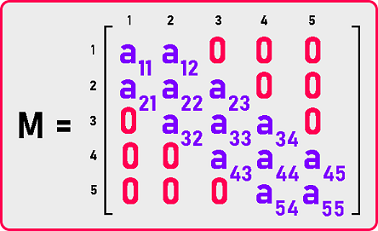Here we have an example of a 5×5 matrix and it’s a tridiagonal matrix.

##### What does it mean by a tridiagonal matrix?

If we look at the elements, non-zero elements are present in the main diagonal, lower diagonal, and upper diagonal and the rest of the elements are all zeros. Let us define the property of this matrix based on indices of the matrix. If we observe the indices of elements of:

1. Main Diagonal: row number is equal to column number (i = j).
2. Lower Diagonal: row number – column number = 1 (i – j = 1).
3. Upper Diagonal: row number – column number = -1 (i – j = -1).

So, the conditions are: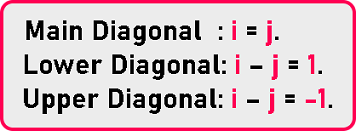They must be non-zero and all other elements must be zero. We can combine these three conditions and write them as: |i – j| <= 1. That is the absolute value of (i – j) is less than or equal to 1 then those elements must be non-zero. Now we can say that: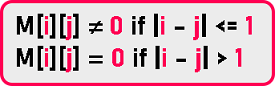##### How many non-zero elements are there?

= n + n-1 + n-1.

= 3n – 2 non-zero elements are there.

We have been trying to avoid storing zero elements. We will avoid storing zero so we’ll store only non-zero elements then we will not be taking a two-dimensional array for this matrix. We will take a single array. What should be the size of that single dimension array? Depends on the number of non-zero elements.

= 3n – 2

= 3 * 5 – 2

= 13

We need 13 spaces for storing these elements. So let us see how to represent this tridiagonal matrix in a single dimension array. As we can see this matrix is not having a uniform number of elements by row or by column. We can represent them diagonal by diagonal.

So let us store the elements diagonal by diagonal. We can pick up either the upper diagonal and main then lower or first lower than main and then upper. So let us store first lower diagonal elements. We will store lower diagonal elements a21, a32, a43, and a54 as: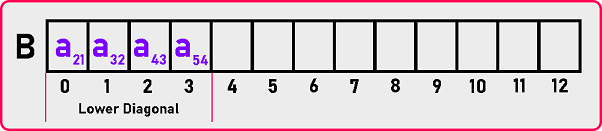Main Diagonal elements a11, a22, a33, a44 and a55 as: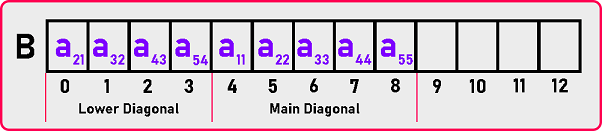Upper diagonal elements a12, a23, a34, and a45 as: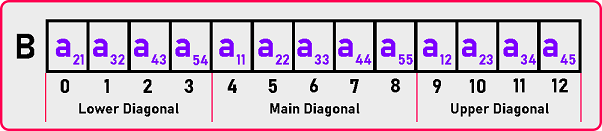##### Now how to map these?

There must be some formula, we cannot come up with a single formula for all the diagonals. We have to handle them separately. Simply we go diagonal by diagonal.

Index (M [i][j]):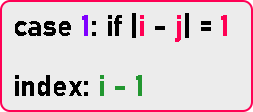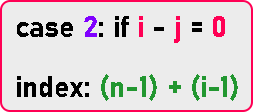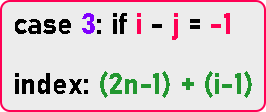Above 3 are required formulas to map matrix elements in a single dimension array with diagonal by diagonal. Let see the code part.

##### TriDiagonal and TriBand Matrix Code in C Language:
```#include <stdio.h>
#include <stdlib.h>

struct Matrix
{
int *B;
int n;
};

void Set (struct Matrix *m, int i, int j, int y)
{
if (i - j == 1)
{
m->B[i - 1] = y;
}
if (i - j == 0)
{
m->B[m->n - 1 + i - 1] = y;
}
if (i - j == -1)
{
m->B[(2 * m->n) - 1 + i - 1] = y;
}
}

int Get (struct Matrix m, int i, int j)
{
if (i - j == 1)
{
return m.B[i - 1];
}
if (i - j == 0)
{
return m.B[m.n - 1 + i - 1];
}
if (i - j == -1)
{
return m.B[(2 * m.n) - 1 + i - 1];
}

return 0;
}

void Display (struct Matrix m)
{
int i, j;

printf ("\nMatrix is: \n");

for (i = 1; i <= m.n; i++)
{
for (j = 1; j <= m.n; j++)
{
if (i - j == 1)
{
printf ("%d ", m.B[i - 1]);
}
else if (i - j == 0)
{
printf ("%d ", m.B[m.n - 1 + i - 1]);
}
else if (i - j == -1)
{
printf ("%d ", m.B[(2 * m.n) - 1 + i - 1]);
}
else
{
printf ("0 ");
}
}
printf ("\n");
}
}

int main ()
{
struct Matrix M;
int i, j, y;

printf ("Enter Dimension of Matrix: ");
scanf ("%d", &M.n);
M.B = (int *) malloc ((3 * M.n - 2) * sizeof (int));

printf ("Enter all the elements of the matrix:\n");
for (i = 1; i <= M.n; i++)
{
for (j = 1; j <= M.n; j++)
{
scanf ("%d", &y);
Set (&M, i, j, y);
}
}

Display (M);
}
```
###### Output: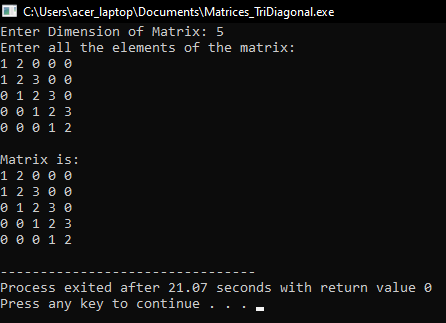In the next article, I am going to discuss Toeplitz Matrix in C Language with Examples. Here, in this article, I try to explain TriDiagonal and TriBand Matrix in C Language with Examples and I hope you enjoy this TriDiagonal and TriBand Matrix in C Language with Examples article.